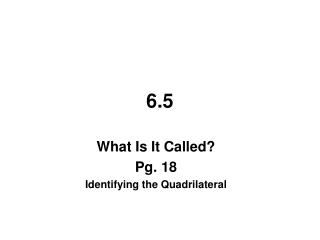DownloadDownload Presentation6.5

# 6.5

Download Presentation## 6.5

- - - - - - - - - - - - - - - - - - - - - - - - - - - E N D - - - - - - - - - - - - - - - - - - - - - - - - - - -
##### Presentation Transcript

1. 6.5 What Is It Called? Pg. 18 Identifying the Quadrilateral

2. 6.5 – What Is It Called? _ Identifying the Quadrilateral In this chapter you have studied many different types of quadrilaterals. Use the properties you have learned to answer the questions in this section. Be careful to not ASSuME anything!

3. 6.24 – NAME THE SHAPE Give the most exact name for the shape. rhombus square kite

4. parallelogram trapezoid parallelogram

5. square rectangle rhombus

6. 6.25 – TRUE OR FALSE? Determine if the following statements are true or false. If false, provide a counterexample.

7. If a quadrilateral is equilateral, then it is equiangular. false rhombus • If a quadrilateral is equiangular, then it is equilateral. false rectangle

8. c. If a quadrilateral is a square, then it is a rectangle. true • d. If a quadrilateral is a rectangle, then it is a square false Not all sides equal

9. e. If the diagonals of a quadrilateral are perpendicular, then it is a rhombus. false kite • f. If the quadrilateral is a rhombus, then its diagonals are perpendicular. true

10. g. If a quadrilateral has one pair of opposite sides parallel, then it is a parallelogram. false trapezoid • h. If a quadrilateral has consecutive sides congruent, then it is a rhombus. false kite

11. Both opposite sides are parallel Both opposite sides are equal Both opposite angles are equal All same-side interior angles are supplementary y x y x Diagonals bisect each other

12. Rhombus: 4 equal sides Diagonals are perpendicular Diagonals bisect angles

13. 4 right angles Diagonals are equal

14. 4 right angles and 4 = sides

15. Consecutive sides are = Diagonals are perpendicular One pair of opposite angles are equal

16. One pair of opposite sides parallel y a x b Two consecutive interior angles are supplementary

17. One opposite side is equal Both base angles are equal A B Diagonals are equal D C

18. 6.26 – VENN DIAGRAMS Use your knowledge of shapes to complete the following Venn Diagrams. Use each shape only once.

19. Mark which shapes have the following properties. 1. Both pairs of opposite sides are parallel.

20. Mark which shapes have the following properties. 2. Both pairs of opposite sides are congruent

21. Mark which shapes have the following properties. 3. Both pairs of opposite angles are congruent

22. Mark which shapes have the following properties. 4. Exactly one pair of opposite sides are parallel

23. Mark which shapes have the following properties. 5. Exactly one pair of opposite sides are congruent

24. Mark which shapes have the following properties. 6. Exactly one pair of opposite angles are congruent

25. Mark which shapes have the following properties. 7. Diagonals are congruent

26. Mark which shapes have the following properties. 8. Diagonals are perpendicular

27. Class Activity • Make a square • Make a rhombus w/ no right angles • Make a rectangle • Make a parallelogram w/ no right angles • Make a kite • Make a trapezoid • Make an isosceles trapezoid • Make an isosceles triangle • Make a equilateral triangle